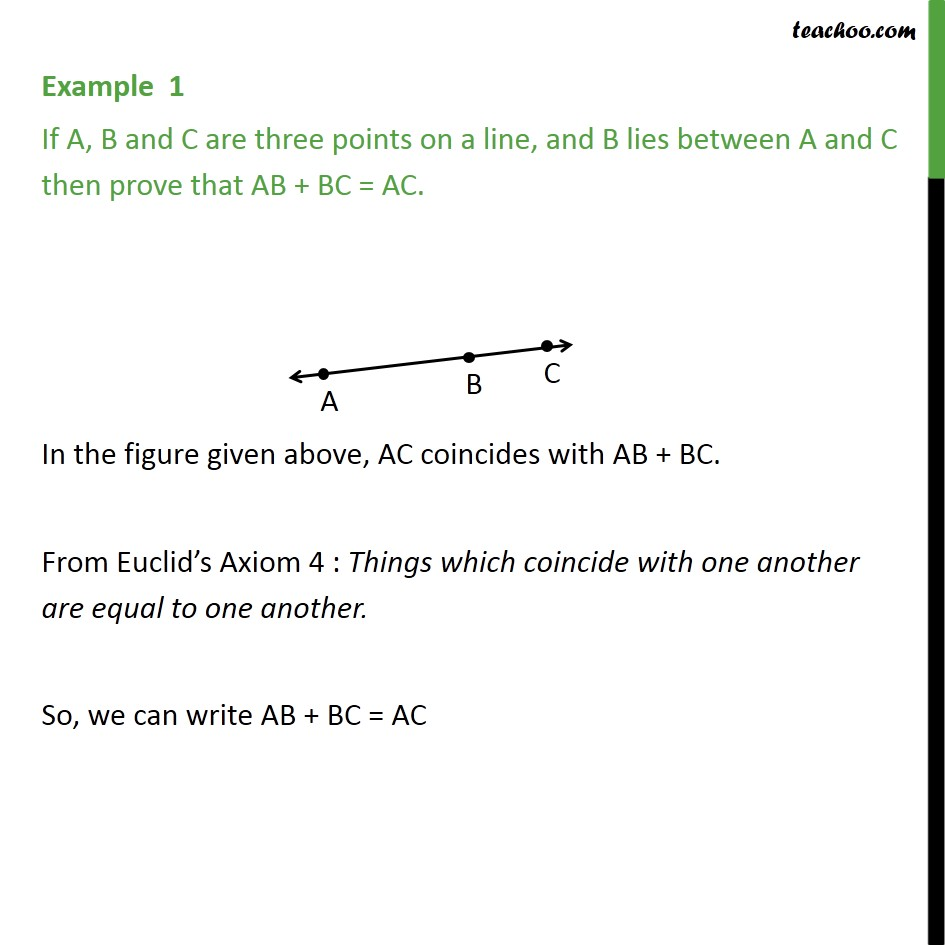Examples

Chapter 5 Class 9 Introduction to Euclid's Geometry
Serial order wiseLearn in your speed, with individual attention - Teachoo Maths 1-on-1 Class

### Transcript

Example 1 If A, B and C are three points on a line, and B lies between A and C then prove that AB + BC = AC. In the figure given above, AC coincides with AB + BC. From Euclid’s Axiom 4 : Things which coincide with one another are equal to one another. So, we can write AB + BC = AC